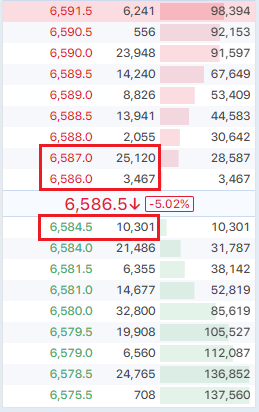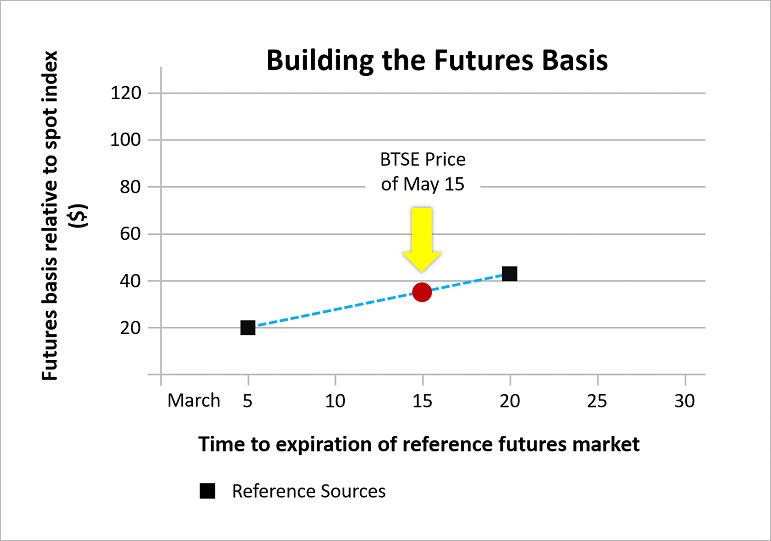Index Price is an important reference when you are investing. It's the average market price of cryptocurrencies on major exchanges. It’s also the primary component of the mark price.

Mark Price is the price used for mark-to-market PnL calculation and platform liquidation, partial liquidation, or forced market buy/sell; Mark Price is designed to be fair and manipulation resistant.

Perpetual Futures Index Price

BTSE Perpetual Futures Index Price = Average Spot Liquidity Mid Price of Major Exchanges.

• Spot Liquidity Mid Price = (Bid Price x Ask Size + Ask Price x Bid Size) / (Bid Size + Ask Size)

Reference values are provided by the following 4 exchanges: Bitfinex, Binance, Houbi and Coinbase Pro.

• In order to ensure the stability of the index price, the average value will be calculated after removing the highest and lowest reference values.
• The reference feeds corresponding to each currency may be different.

* When there are 5 reference values, remove the highest and lowest values, then calculate the average of the remaining 3 values.

Perpetual Futures Mark Price

BTSE Perpetual Futures Mark Price = (Spot Liquidity Mid Price x 90% + BTSE Impact Mid Price x 10%)

• Spot Liquidity Mid Price = (Bid Price x Ask Size + Ask Price x Bid Size) / (Bid Size + Ask Size)
• BTSE Impact Mid Price = Average (Impact Bid Price, Impact Ask Price)
• Impact Bid Price: The average buy price of the first 10,000 highest bid orders in the Order Book
• Impact Ask Price: The average sell price of the first 10,000 lowest ask orders in the Order Book

For example:
In the following order book:
- Impact Bid Price = (6,584.5 x 10,000) / 10,000 = 6,584.5
- Impact Ask Price = (6,586 x 3,467 + 6,587 x 6,533) / 10,000 = 6,586.65
- Impact Mid Price = (6,586.65 + 6,584.5) / 2 = 6,585.58Perpetual futures mark price enabling conditions:

• The spread between mark price and BTSE perpetual futures liquidity mid price cannot exceed 2%
• When the spread ≥ 2%, Mark Price = Index Price

Time-Based Futures Index Price

BTSE Time-based Futures Index Price = Spot Liquidity Mid Price x (1 + Fair Basis)

• Spot Liquidity Mid Price = (Best Bid Price x Ask Size + Best Ask Price x Bid Size) / (Bid Size + Ask Size)
• Fair Basis = Average Time-based Futures Premium (or Discount) Percentage of major exchanges.

Time-based Futures Premium (or Discount) Percentage:

• When the expiration date of BTSE's time-based futures is the same as the reference exchanges:
Calculate the average premium (or discount) percentage of the reference exchanges.
• When the expiration date of BTSE's time-based futures is different from the reference exchanges:
Take two reference sources with the closest expiration dates, then use interpolation (or extrapolation) to get the premium (or discount) percentage.

For example:
If a BTSE contract’s expiration date is 03/15, and the closest reference sources are 03/05 and 03/20.
Since 03/15 is in between the reference sources, we use interpolation to calculate the premium (or discount) percentage.On the contrary, if the contract’s expiration date is 03/25, and the closest reference sources are 03/05 and 03/20.
Since 03/25 is outside of the reference sources, we use extrapolation to calculate the premium (or discount) percentage.• Premium (or discount) percentage enabling conditions:
• The reference premium (or discount) percentage must not be stale
• After weighting, the index spread should not exceed 0.5%
• When the above conditions are not met, premium (or discount) percentage = 0

Reference values are provided by the following 4 exchanges: Bitfinex, Binance, Houbi and Coinbase Pro.

• In order to ensure the stability of the index price, the average value will be calculated after removing the highest and lowest reference values.
• The reference feeds corresponding to each currency may be different. For more details, please see here.

* When there are 5 reference values, remove the highest and lowest values, then calculate the average of the remaining 3 values.

Time-Based Futures Mark Price

BTSE Time-based Futures Mark Price = BTSE Time-based Futures Index Price x 90% + Liquidity Mid Price of BTSE Time-base Futures Market x 10%

• BTSE Time-based Futures Index Price: Please refer to the Time-based Futures Index Price section
• Liquidity Mid Price of BTSE Time-base Futures Market = (Bid Price x Ask Size + Ask Price x Bid Size) / (Bid Size + Ask Size)

Time-based futures mark price enabling conditions:

• The spread between mark price and BTSE time-based futures liquidity mid price cannot exceed 2%
• When the spread ≥ 2%, Mark Price = Index Price